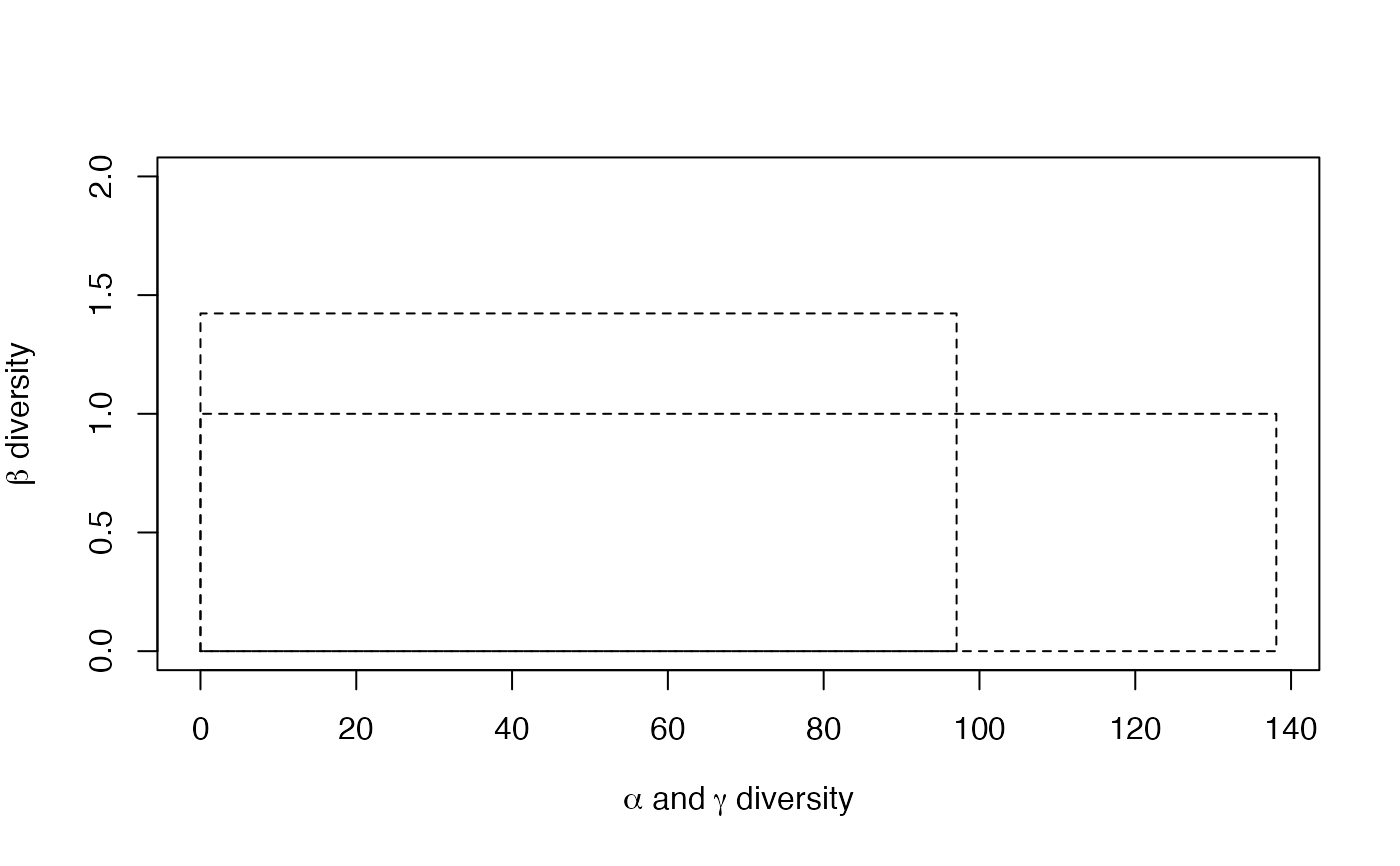Partitions the diversity of a metacommunity into alpha and beta components.

DivPart(q = 1, MC, Biased = TRUE, Correction = "Best", Tree = NULL,
Normalize = TRUE, Z = NULL, CheckArguments = TRUE)
is.DivPart(x)
# S3 method for DivPart
plot(x, ...)
# S3 method for DivPart
autoplot(object, col = ggplot2::GeomRect$default_aes$fill,
border = ggplot2::GeomRect$default_aes$colour, ...)
# S3 method for DivPart
summary(object, ...)

## Arguments

q

A number: the order of diversity. Default is 1.

MC

A MetaCommunity object.

Biased

Logical; if FALSE, a bias correction is appplied.

Correction

A string containing one of the possible corrections.

The correction must be accepted by AlphaEntropy, BetaEntropy and GammaEntropy. "Best" is the default value.

Tree

An object of class hclust, phylo, phylog or PPtree. The tree must be ultrametric.

Normalize

If TRUE (default), diversity is not affected by the height of the tree.
If FALSE, diversity is proportional to the height of the tree.

Z

A relatedness matrix, i.e. a square matrix whose terms are all positive, strictly positive on the diagonal. Generally, the matrix is a similarity matrix, i.e. the diagonal terms equal 1 and other terms are between 0 and 1.

CheckArguments

Logical; if TRUE, the function arguments are verified. Should be set to FALSE to save time when the arguments have been checked elsewhere.

x

An object to be tested or plotted.

object

A MCdiversity object to be summarized or plotted.

col

The color used to fill the bars. See "Color Specification" in par.

border

The color of the borders around the bars. See rect.

...

Additional arguments to be passed to the generic methods.

## Details

DivPart partitions the diversity of the metacommunity into alpha and beta components. It supports estimation-bias correction.

If Tree is provided, the phylogenetic diversity is calculated else if Z is not NULL, then similarity-based entropy is calculated.

Beta diversity/entropy is calculated from Gamma and Alpha when bias correction is required, so community values are not available.

## Value

A DivPart object. It is a list:

MetaCommunity

The name of the MetaCommunity object containing inventory data.

Order

The value of q.

Biased

Logical. If FALSE, bias corrected values of diversity have been computed.

Correction

The estimation bias correction used to calculate diversity.

Method

The method used to calculate entropy ("HCDT", "Similarity-based").

Tree

The phylogenetic or functional tree used to calculate phylodiversity.

Normalized

Logical. Indicates whether phylodiversity is normalized or proportional to the height of the tree.

Z

The matrix used to calculate similarity-based entropy.

The alpha diversity of communities.

The beta diversity of communities.

The gamma diversity of the metacommunity.

A vector containing the alpha diversity of each community.

TotalAlphaEntropy

The alpha entropy of communities.

TotalBetaEntropy

The beta entropy of communities.

GammaEntropy

The gamma entropy of the metacommunity.

CommunityAlphaEntropies

A vector containing the alpha entropy of each community.

DivPart objects can be summarized and plotted.

## Author

Eric Marcon <Eric.Marcon@agroparistech.fr>, Bruno Herault <Bruno.Herault@cirad.fr>

DivProfile

## Examples

# Load Paracou data (number of trees per species in two 1-ha plot of a tropical forest)
data(Paracou618)
# Estimate Shannon diversity.
summary(DivPart(q = 1, Paracou618.MC, Biased = FALSE) -> dp)
#> HCDT diversity partitioning of order 1 of metaCommunity Paracou618.MC
#>  with correction: UnveilJ
#> Alpha diversity of communities:
#>     P006     P018
#>  83.7268 118.2713
#> Total alpha diversity of the communities:
#>  97.06467
#> Beta diversity of the communities:
#>  UnveilJ
#> 1.422843
#> Gamma diversity of the metacommunity:
#>  UnveilJ
#> 138.1078
plot(dp)